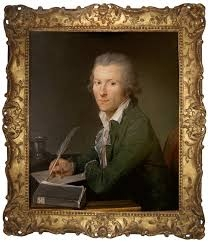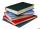# Unknown number

4/5 of a number is 276. what is 2/3 of the same number?

x =  230

### Step-by-step explanation:Did you find an error or inaccuracy? Feel free to write us. Thank you!Tips to related online calculators
Need help to calculate sum, simplify or multiply fractions? Try our fraction calculator.

## Related math problems and questions:

• Unknown numberIf I reduced the sum of the numbers 70 and the unknown number three times, I would get 100. what is the unknown number?
• Unknown xIf we add to unknown number 21, then divide by 6 and then subtract 51, we get back an unknown number. What is this unknown number?
• Unknown numberFind the unknown number equal to a quarter of a fifth of a number, which is by 152 more than an unknown number.
• Unknown numberSamuel wrote unknown number. Then he had add 200000 to the number and the result multiply by three. When it calculated he was surprised, because the result would have received anyway, if write digit to the end of original number. Find unknown number.
• Unknown number 5I think of an unknown number. If we enlarge it five times then subtract 3 and the result decreases by 75% we get one greater than the number. What number am I thinking of?
• The quotientThe quotient of g and 55 is the same as 279. What is g?
• Numbers divisionWith what number should be divided mixed number 2 3/4 to get 11/12?
• Janka and DankaJanka and Danka began to read the same day the books. Janka had 276 pages, Danka 204 pages. They agreed that both read every day the same number of pages until theirs books read. A) How many maximum pages they had to read the day to honor their agreement?
• RemaindersIt is given a set of numbers { 170; 244; 299; 333; 351; 391; 423; 644 }. Divide this numbers by number 66 and determine set of remainders. As result write sum of this remainders.
• Fractions and mixed numerals(a) Convert the following mixed numbers to improper fractions. i. 3 5/8 ii. 7 7/6 (b) Convert the following improper fraction to mixed number. i. 13/4 ii. 78/5 (c) Simplify these fractions to their lowest terms. i. 36/42 ii. 27/45 2. evaluate following ex
• Unknown number 6Determine the unknown number, which is by 1.5 greater than its fourth.
• ExpressionsLet k represent an unknown number, express the following expressions: 1. The sum of the number n and two 2. The quotient of the numbers n and nine 3. Twice the number n 4. The difference between nine and the number n 5. Nine less than the number n
• Result and remainderAfter dividing the unknown number by the number 23, the quotient 11 and the remainder 4 are formed. Find an unknown number.
• Sports studentsThere are 120 athletes, 48 volleyball players, and 72 handball players at the school with extended sports training. Is it possible to divide sports students into groups so that the number in each group is the same and expressed by the largest possible num
• Find unknown numberWhat is the number between 50 and 55 that is divisible by 2,3,6,9?
• Four multiplesFour multiples of 6 he writes the following 12  24  56  72, which is correct?
• Guess numberWith what number should be divided, number 9727 to be reduced by 70%?